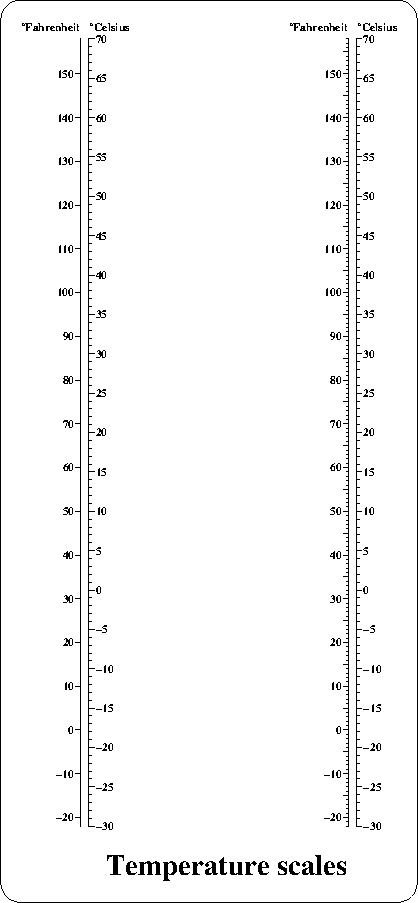# plaxes

## plots x- and/or y-axes and scales the user units

parametertypeunitsdescription
xleft,ybotfloatuuvalue at the left/low end of the axes
xright,ytopfloatuuvalue at the right/high end of the axes
xlen,ylenfloatmmlengths of the axes
xtext,ytextchar*--strings plotted at the right/upper end of the axes
textchar*--string plotted at the left end of the x-axis
returns:void

## Description

plaxes plots x- and y-axes and sets scale factors and user origin The placement of axes and numbers along the axes is governed by global variables set by plset (T=TRUE, F=FALSE):
 global default value description \b X/YIN F/F T number at the right/above of the x/y axis F at the left/under X/YSQUAR T/F T number square to the x/y-axis F number in the direction of the x/y-axis X/YANGLE 0/90 val directions of the axes relative to the initial x-axis; any angle is permitted CROSS F T axes cross at user unit set by X/YCROSS F axes cross at xleft,ybot X/YCROSS xleft/ybot val y/x axis crosses the x/y axis at val user units if CROSS has been set to a non-zero value X/YMARK 0/0 val x/y axis scale marks are placed every val user units 0 x/y axis scale marks are placed automatically X/YSKIP 0 val x/y axis numbers are placed at every val scale mark 0 x/y axis numbers are placed automatically X/YGRID F T grid lines at plotted through the numbered scale marks of the y/x axis F no grid lines are plotted

## Bugs

Plaxes plots in GXcopy mode, even if the user has set another mode.

## Examples

several features: plotting of a single axis by setting xl=0 or xleft=xright, plotting of scale ticks and number to the left or to the right of the axis, and the usage of YMARK:
```#include <simplot.h>
#include <stdio.h>
int main() {
int i,Fmin=-22,Fmax=158,Cmin=-30,Cmax=70;

plinit(PS,"",A4,50,50,"TB","");

for (i=0;i<=66;i+=66) {
plmvorgm(i,0);
// note that we need tricks to put the Celsius and the Fahrenheit
// on the correct side. Celsius is put above the Fahrenheit axis
// and vice versa, and spaces are used for shifting

plaxes(0,Fmin,0,Fmax,0,200,"","   #0#Celsius","");
// plots vertical scale; x-axis not used
plset(YIN,TRUE);
// numbers will be plotted "inside", that is: right of y-axis
plmvorgm(2,0);
// moves origin 2 mm to the right
plaxes(0,Cmin,0,Cmax,0,200," ","#0#Fahrenheit   "," ");
// plots celsius scale 2 mm right of fahrenheit scale
plset(YIN,FALSE);
// resets plotting 'outside' that is: left of y-axis
plset(YMARK,TRUE);
// causes plotting of scale marks at each degree
}
plset(HEIGHT,5);
plotm(-33,-10,UP);
plformat(0,0,"Temperature scales");
plframe(5,5);
exit(0);
}
```plpoly plarc plfont plpline plhist plarcr plformat plloop plmvorg simplot program plfunc plpolar plrotate pltrace plpolv
• Home